# Image classification from scratch

vision
Training an image classifier from scratch on the Kaggle Cats vs Dogs dataset.
Authors

## Introduction

This example shows how to do image classification from scratch, starting from JPEG image files on disk, without leveraging pre-trained weights or a pre-made Keras Application model. We demonstrate the workflow on the Kaggle Cats vs Dogs binary classification dataset.

We use the `image_dataset_from_directory` utility to generate the datasets, and we use Keras image preprocessing layers for image standardization and data augmentation.

## Setup

``````library(tensorflow)
library(keras)
library(tfdatasets)
set.seed(1234)``````

## Load the data: the Cats vs Dogs dataset

``````url <- "https://download.microsoft.com/download/3/E/1/3E1C3F21-ECDB-4869-8368-6DEBA77B919F/kagglecatsanddogs_5340.zip"
options(timeout = 60 * 5) # 5 minutes
## To see a list of everything in the zip file:
# zip::zip_list("kagglecatsanddogs_5340.zip") |> tibble::as_tibble()
zip::unzip("kagglecatsanddogs_5340.zip")``````

Now we have a `PetImages` folder which contain two subfolders, `Cat` and `Dog`. Each subfolder contains image files for each category.

``fs::dir_info("PetImages")``
``````# A tibble: 2 × 18
path         type   size permiss…¹ modification_time   user  group devic…²
<fs::path>   <fct> <fs:> <fs::per> <dttm>              <chr> <chr>   <dbl>
1 …tImages/Cat dire…  272K rwx------ 2023-02-11 18:23:59 toma… toma…   66306
2 …tImages/Dog dire…  272K rwx------ 2023-02-11 18:23:59 toma… toma…   66306
# … with 10 more variables: hard_links <dbl>, special_device_id <dbl>,
#   inode <dbl>, block_size <dbl>, blocks <dbl>, flags <int>,
#   generation <dbl>, access_time <dttm>, change_time <dttm>,
#   birth_time <dttm>, and abbreviated variable names ¹​permissions,
#   ²​device_id``````
``fs::dir_info("PetImages", recurse = TRUE)``
``````# A tibble: 23,202 × 18
path       type    size permiss…¹ modification_time   user  group devic…²
<fs::path> <fct> <fs::> <fs::per> <dttm>              <chr> <chr>   <dbl>
1 …mages/Cat dire…   272K rwx------ 2023-02-11 18:23:59 toma… toma…   66306
2 …Cat/0.jpg file   11.9K rw------- 2017-02-10 09:54:54 toma… toma…   66306
3 …Cat/1.jpg file   16.5K rw------- 2017-02-10 09:54:54 toma… toma…   66306
4 …at/10.jpg file   34.5K rw------- 2017-02-10 09:54:54 toma… toma…   66306
5 …t/100.jpg file     30K rw------- 2017-02-10 09:54:54 toma… toma…   66306
6 …/1000.jpg file   25.7K rw------- 2017-02-10 09:54:54 toma… toma…   66306
7 …10000.jpg file  127.2K rw------- 2017-02-10 09:54:54 toma… toma…   66306
8 …10001.jpg file   26.4K rw------- 2017-02-10 09:54:54 toma… toma…   66306
9 …10002.jpg file   25.6K rw------- 2017-02-10 09:54:54 toma… toma…   66306
10 …10003.jpg file   27.9K rw------- 2017-02-10 09:54:54 toma… toma…   66306
# … with 23,192 more rows, 10 more variables: hard_links <dbl>,
#   special_device_id <dbl>, inode <dbl>, block_size <dbl>, blocks <dbl>,
#   flags <int>, generation <dbl>, access_time <dttm>, change_time <dttm>,
#   birth_time <dttm>, and abbreviated variable names ¹​permissions,
#   ²​device_id``````

### Filter out corrupted images

When working with lots of real-world image data, corrupted images are a common occurrence. Let’s filter out badly-encoded images that do not feature the string “JFIF” in their header.

``````n_deleted <- 0L
for(filepath in list.files("PetImages", pattern = "\\.jpg\$",
recursive = TRUE, full.names = TRUE)) {
n_deleted <- n_deleted + 1L
}
}

cat(sprintf("Deleted %d images\n", n_deleted))``````
``Deleted 0 images``

## Generate a `Dataset`

``````image_size <- c(180, 180)
batch_size <- 32

train_ds <- image_dataset_from_directory(
"PetImages",
validation_split = 0.2,
subset = "training",
seed = 1337,
image_size = image_size,
batch_size = batch_size,
)
val_ds <- image_dataset_from_directory(
"PetImages",
validation_split = 0.2,
subset = "validation",
seed = 1337,
image_size = image_size,
batch_size = batch_size,
)``````

## Visualize the data

``````batch <- train_ds %>%
as_iterator() %>%
iter_next()

str(batch)``````
``````List of 2
\$ :<tf.Tensor: shape=(32, 180, 180, 3), dtype=float32, numpy=…>
\$ :<tf.Tensor: shape=(32), dtype=int32, numpy=…>``````
``c(images, labels) %<-% batch``
``````display_image_tensor <- function(x, ..., max = 255,
plot_margins = c(0, 0, 0, 0)) {
if(!is.null(plot_margins))
par(mar = plot_margins)

x %>%
as.array() %>%
drop() %>%
as.raster(max = max) %>%
plot(..., interpolate = FALSE)
}

par(mfrow = c(3, 3))
for (i in 1:9)
display_image_tensor(images[i,,,],
plot_margins = rep(.5, 4))``````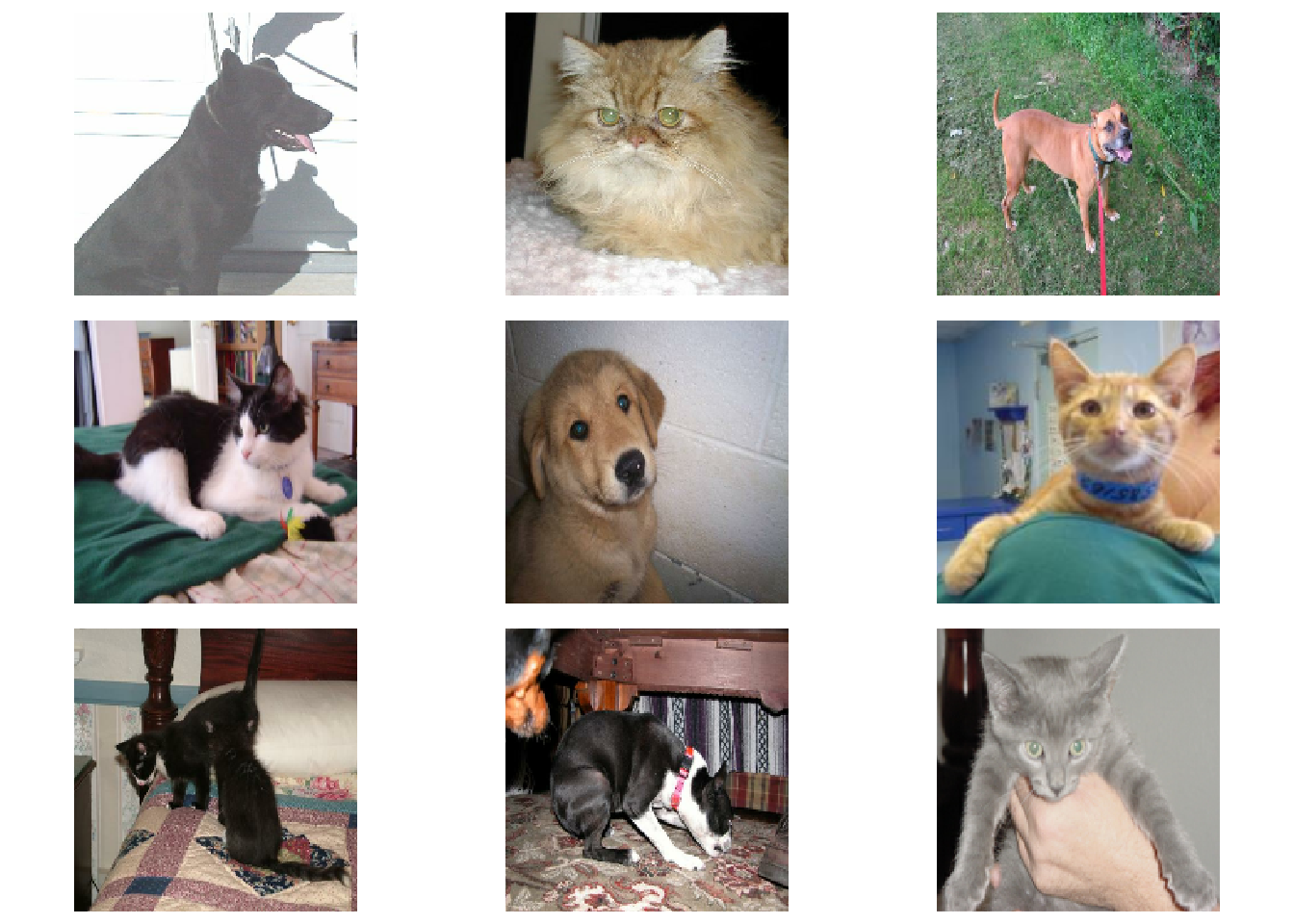## Using image data augmentation

When you don’t have a large image dataset, it’s a good practice to artificially introduce sample diversity by applying random yet realistic transformations to the training images, such as random horizontal flipping or small random rotations. This helps expose the model to different aspects of the training data while slowing down overfitting.

``````# If you are on an M1 mac, you may need to wrap this model definition in
# with(tf\$device("CPU"), { ... })

data_augmentation <-
keras_model_sequential(input_shape = c(image_size, 3)) %>%
layer_random_flip("horizontal") %>%
layer_random_rotation(factor = 0.1)``````

Let’s visualize what the augmented samples look like, by applying `data_augmentation` repeatedly to the first image in the dataset:

``````par(mfrow = c(3, 3))
for (i in 1:9) {
images[4, , , , drop = FALSE] %>%
data_augmentation() %>%
display_image_tensor()
}``````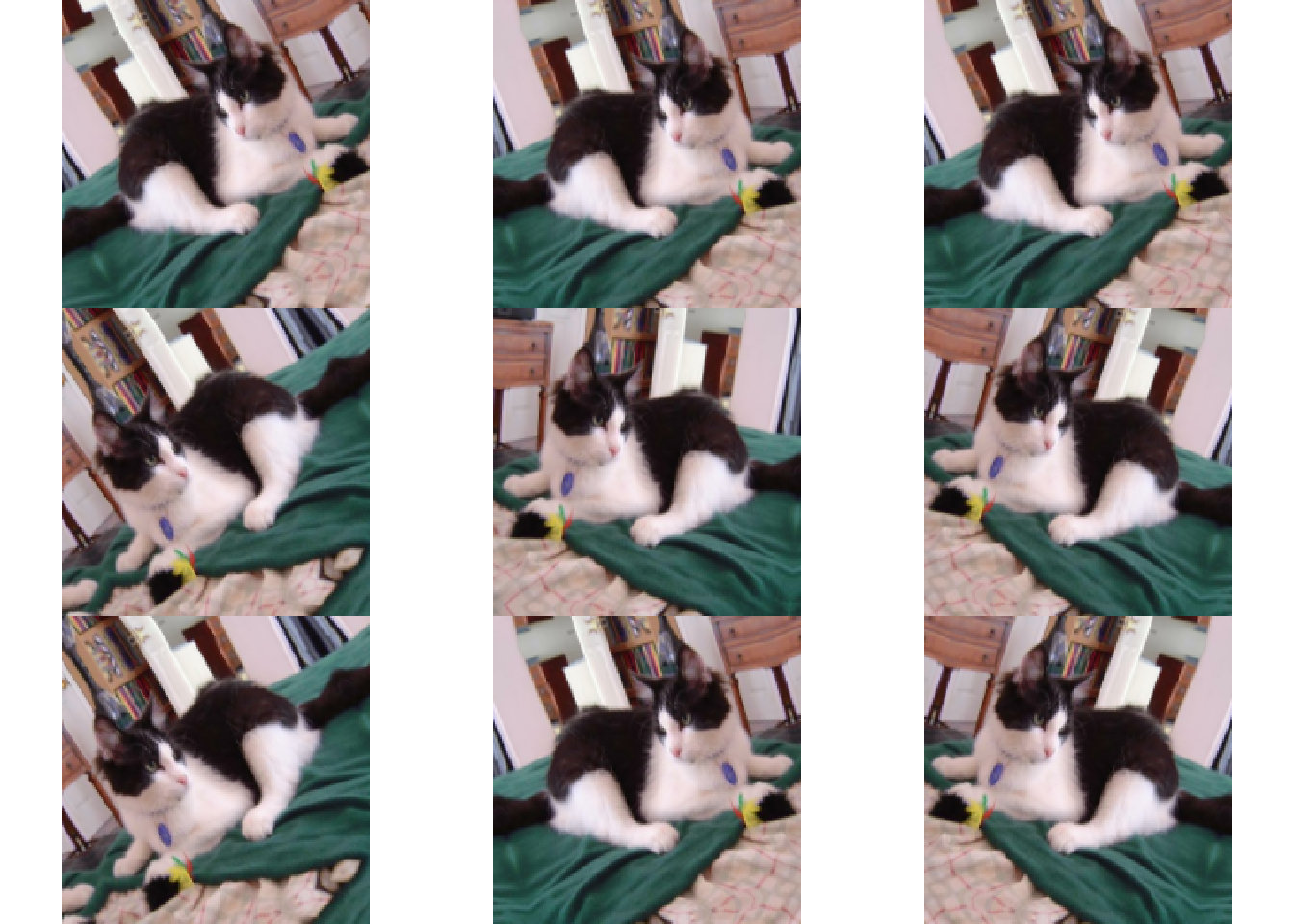## Standardizing the data

Our image are already in a standard size (180x180), as they are being yielded as contiguous `float32` batches by our dataset. However, their RGB channel values are in the `[0, 255]` range. This is not ideal for a neural network; in general you should seek to make your input values small. Here, we will standardize values to be in the `[0, 1]` by using a `Rescaling` layer at the start of our model.

## Two options to preprocess the data

There are two ways you could be using the `data_augmentation` preprocessor: Option 1: Make it part of the model, like this:

``````x <- layer_input(shape = input_shape) %>%
data_augmentation() %>%
layer_rescaling(1./255)
...  # Rest of the model``````

With this option, your data augmentation will happen on device, synchronously with the rest of the model execution, meaning that it will benefit from GPU acceleration.

Note that data augmentation is inactive at test time, so the input samples will only be augmented during `fit()`, not when calling `evaluate()` or `predict()`.

If you’re training on GPU, this may be a good option.

Option 2: apply it to the dataset, so as to obtain a dataset that yields batches of augmented images, like this:

``````augmented_train_ds <- train_ds %>%
dataset_map(function(x, y) {
x <- data_augmentation(x, training = TRUE)
list(x, y)
})``````

With this option, your data augmentation will happen on CPU, asynchronously, and will be buffered before going into the model (this is because all TF Dataset operations, include any defined in `dataset_map()`, are pinned to the CPU).

If you’re training on CPU, this is the better option, since it makes data augmentation asynchronous and non-blocking.

In our case, we’ll go with the second option. If you’re not sure which one to pick, this second option (asynchronous preprocessing) is always a solid choice.

## Configure the dataset for performance

Let’s apply data augmentation to our training dataset, and let’s make sure to use buffered prefetching so we can yield data from disk without having I/O becoming blocking:

``````# Apply `data_augmentation` to the training images.
train_ds <- train_ds %>%
dataset_map(function(images, labels) {
list(data_augmentation(images, training = TRUE),
labels)
})

# Prefetching samples in GPU memory helps maximize GPU utilization.
train_ds %<>% dataset_prefetch()
val_ds   %<>% dataset_prefetch()``````

## Build a model

We’ll build a small version of the Xception network. We haven’t particularly tried to optimize the architecture; if you want to do a systematic search for the best model configuration, consider using KerasTuner.

Note that:

• We start the model with the `data_augmentation` preprocessor, followed by a `Rescaling` layer.
• We include a `Dropout` layer before the final classification layer.
``````make_model <- function(input_shape, num_classes) {

inputs <- layer_input(shape = input_shape)

x <- inputs %>%
# data augmentation() ? %>%
layer_rescaling(1.0 / 255)

x <- x %>%
layer_conv_2d(128, 3, strides = 2, padding = "same") %>%
layer_batch_normalization() %>%
layer_activation("relu")

previous_block_activation <- x  # Set aside residual
for (size in c(256, 512, 728)) {
x <- x %>%
layer_activation("relu") %>%
layer_separable_conv_2d(size, 3, padding = "same") %>%
layer_batch_normalization() %>%

layer_activation("relu") %>%
layer_separable_conv_2d(size, 3, padding = "same") %>%
layer_batch_normalization() %>%

layer_max_pooling_2d(3, strides = 2, padding = "same")

# Project residual
residual <- previous_block_activation %>%
layer_conv_2d(filters = size, kernel_size = 1, strides = 2,

previous_block_activation <- x  # Set aside next residual
}

x <- x %>%
layer_separable_conv_2d(1024, 3, padding = "same") %>%
layer_batch_normalization() %>%
layer_activation("relu") %>%
layer_global_average_pooling_2d()

if (num_classes == 2) {
activation <- "sigmoid"
units <- 1
} else {
activation <- "softmax"
units <- num_classes
}

outputs <- x %>%
layer_dropout(0.5) %>%
layer_dense(units, activation = activation)

return(keras_model(inputs, outputs))
}

model <- make_model(input_shape = c(image_size, 3), num_classes = 2)``````
``plot(model)``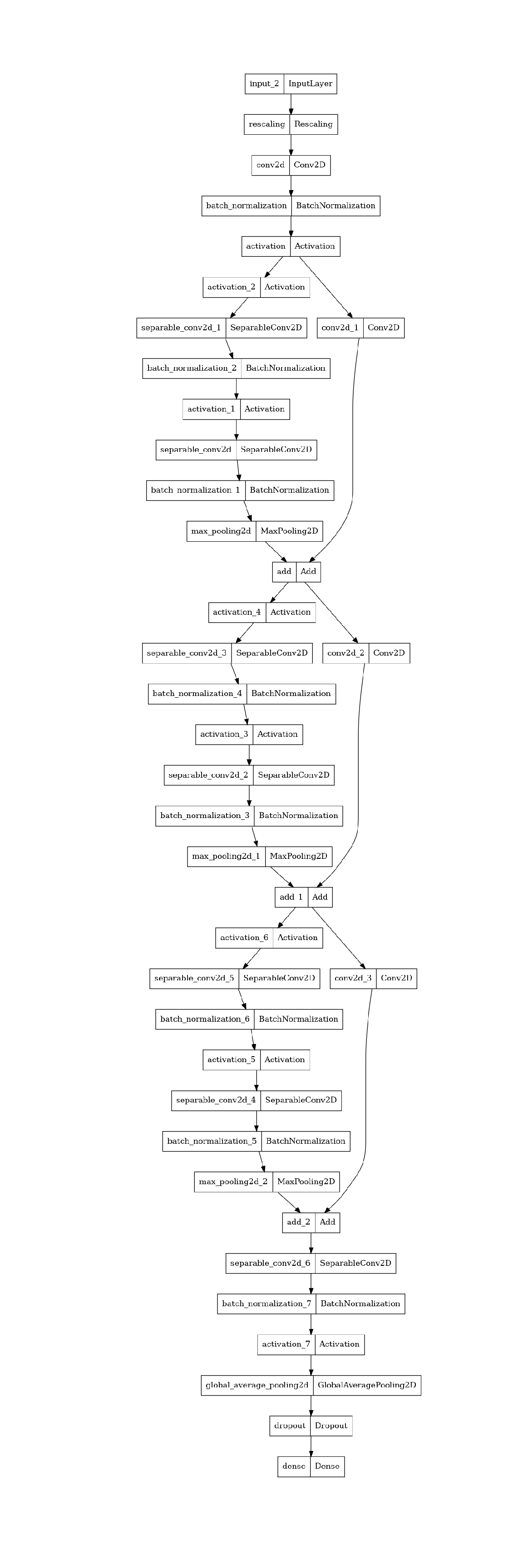``````epochs <- 25

callbacks <- list(callback_model_checkpoint("save_at_{epoch}.keras"))
model %>% compile(
loss = "binary_crossentropy",
metrics = list("accuracy"),
)
history <- model %>% fit(
train_ds,
epochs = epochs,
callbacks = callbacks,
validation_data = val_ds,
)
plot(history)``````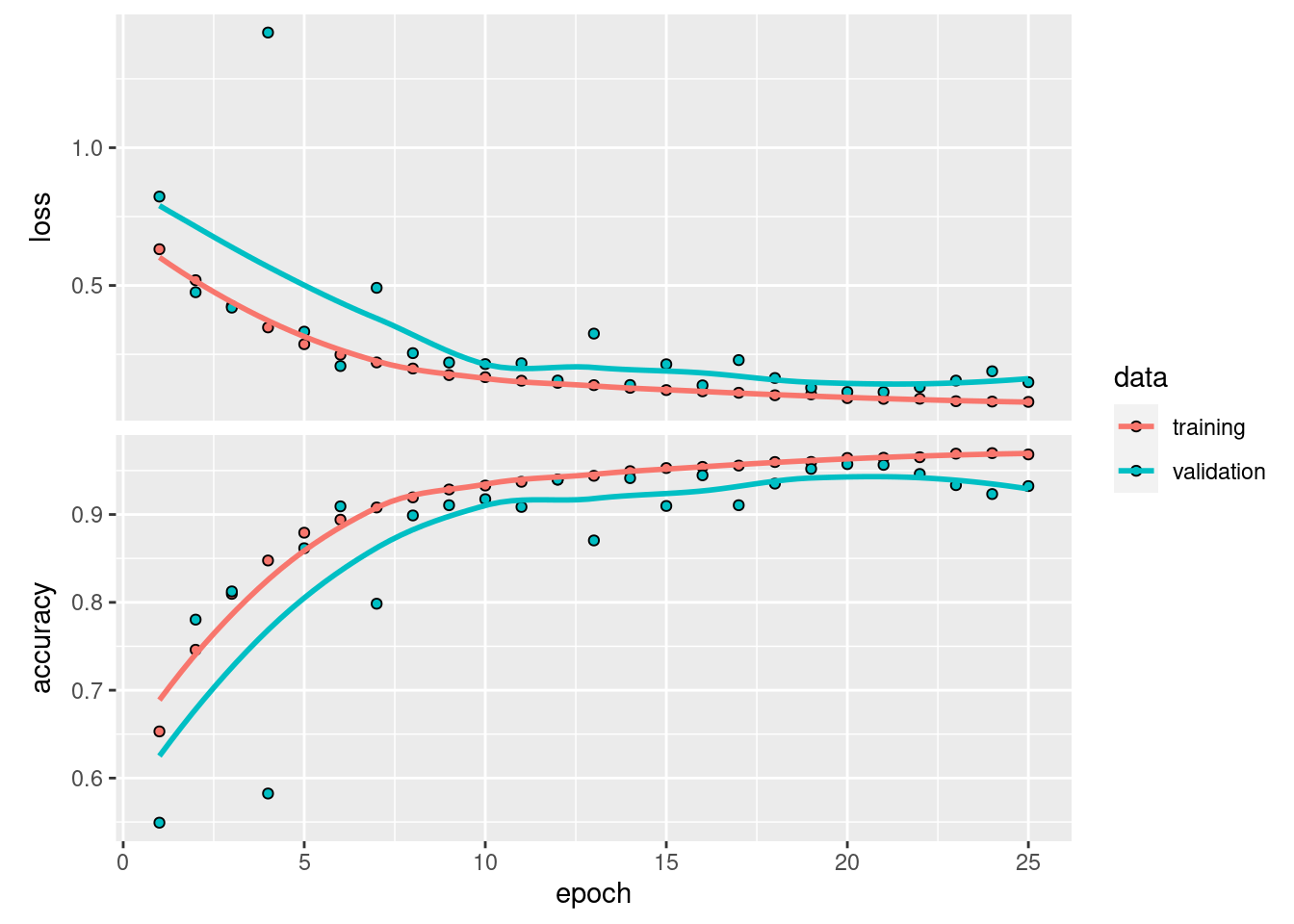We get to >90% validation accuracy after training for 25 epochs on the full dataset (in practice, you can train for 50+ epochs before validation performance starts degrading).

## Run inference on new data

You can reload one of the models saved by the checkpoint callback like this:

``model <- load_model_tf("save_at_25.keras")``

Note that data augmentation and dropout are inactive at inference time.

``````# load an image as a tensor
img_tensor <-
"PetImages/Cat/6779.jpg" %>%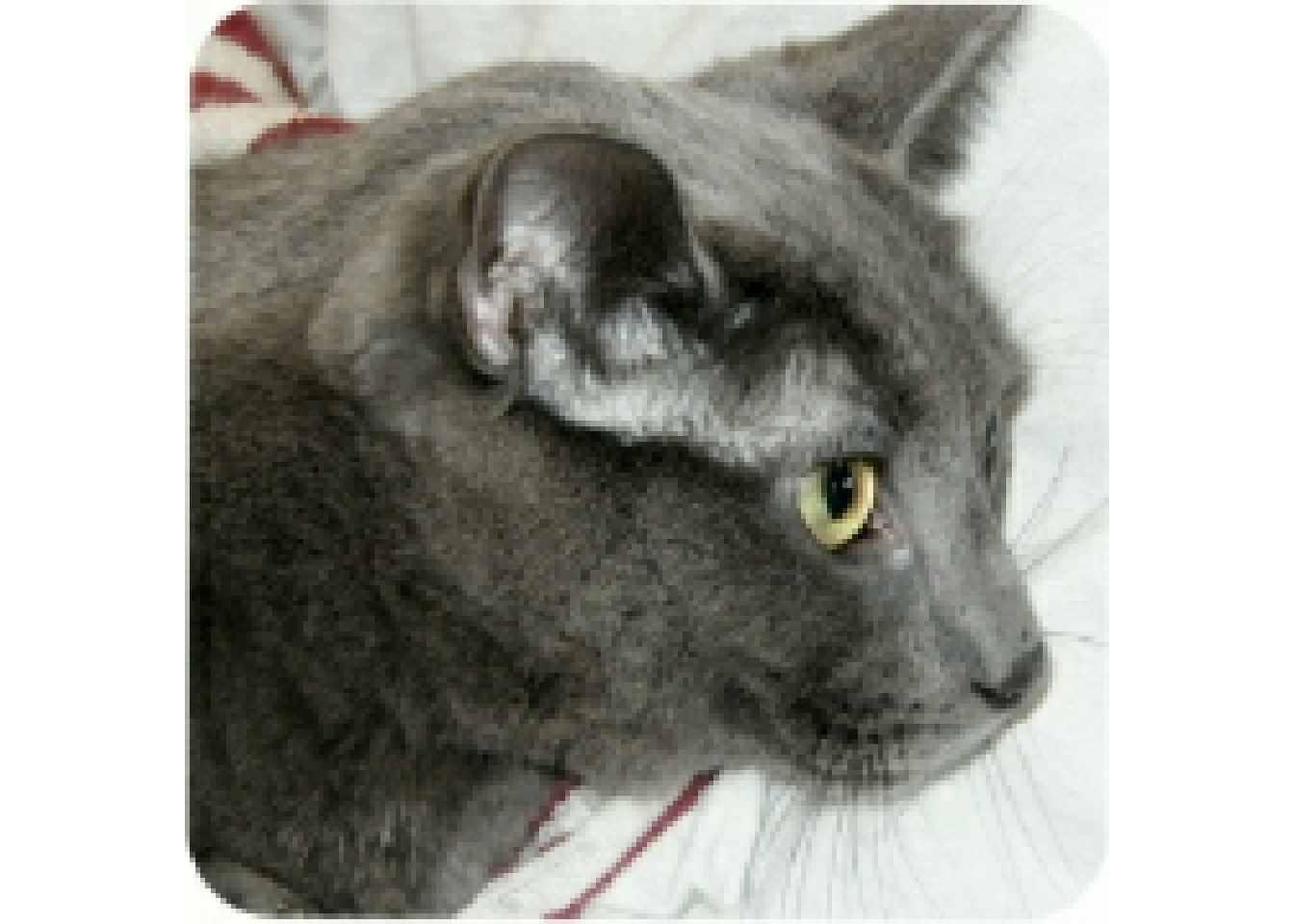``sprintf("This image is %.2f%% cat and %.2f%% dog.", 100 * (1 - score), 100 * score)``
`` "This image is 69.22% cat and 30.78% dog."``